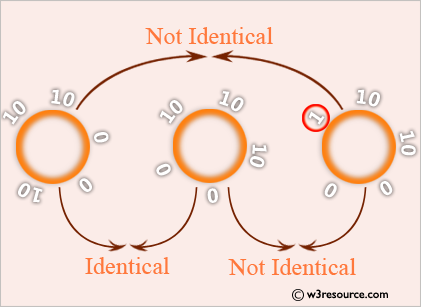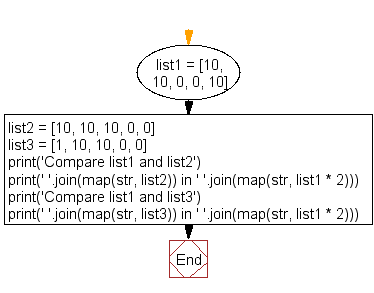﻿ Python: Check whether two lists are circularly identical - w3resource# Python: Check whether two lists are circularly identical

## Python List: Exercise - 26 with Solution

Write a python program to check whether two lists are circularly identical.Sample Solution:-

Python Code:

``````list1 = [10, 10, 0, 0, 10]
list2 = [10, 10, 10, 0, 0]
list3 = [1, 10, 10, 0, 0]

print('Compare list1 and list2')
print(' '.join(map(str, list2)) in ' '.join(map(str, list1 * 2)))
print('Compare list1 and list3')
print(' '.join(map(str, list3)) in ' '.join(map(str, list1 * 2)))
```
```

Sample Output:

```Compare list1 and list2
True
Compare list1 and list3
False
```

Flowchart:## Visualize Python code execution:

The following tool visualize what the computer is doing step-by-step as it executes the said program:

Python Code Editor:

Have another way to solve this solution? Contribute your code (and comments) through Disqus.

What is the difficulty level of this exercise?

Test your Python skills with w3resource's quiz

﻿

## Python: Tips of the Day

Floor Division:

When we speak of division we normally mean (/) float division operator, this will give a precise result in float format with decimals.

For a rounded integer result there is (//) floor division operator in Python. Floor division will only give integer results that are round numbers.

```print(1000 // 300)
print(1000 / 300)```

Output:

```3
3.3333333333333335```